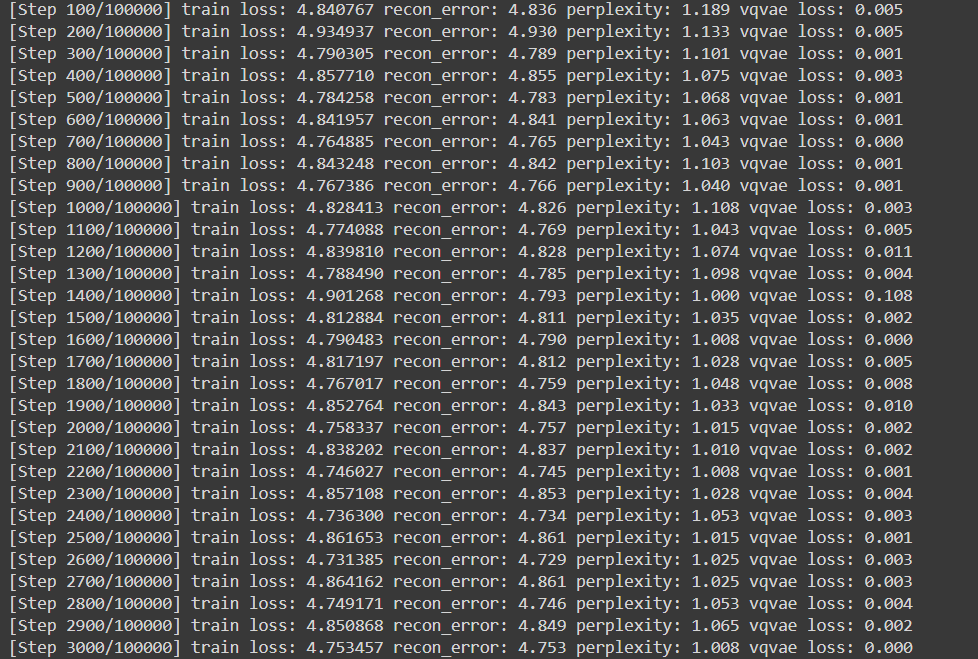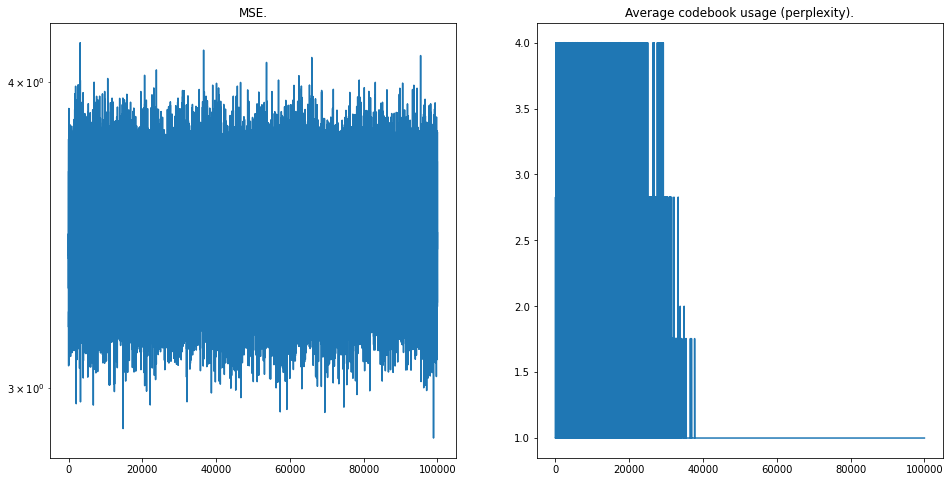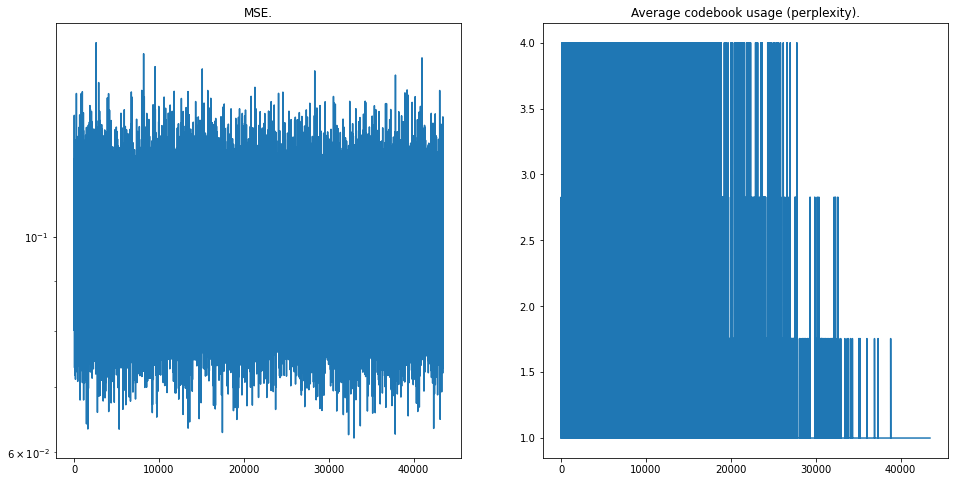$\delta$

# Bug report

June 26, 2021

## the problemBoth losses (i.e. MSE reconstruction loss) bounce around, not moving.

## possible causes

Once cause could be that the training set is too small, so there isn't enough to learn. If this were the case, I think two things would also happen:

• The vqvae reconstructs almost perfectly
• The loss is lower

This leads me to believe that it's not this, though I haven't sampled the model yet.

Another is an issue with the architecture. If one of the values is off, maybe nothing works and its passing through dummy values? Certainly possible.

## what's been tried

Originally, I was using a subset of the gtzan_music_speech dataset for training. This comes with an obvious problem, namely that the model is trying to learn both music and speech. I tried to move to the gtzan dataset, but it was very large. Also, there aren't a lot of samples there (only 1000), so instead I tried to split each 30-second sample into 1-second chunks. I kept crashing the colab GPUs though, probably because I was trying to operate on the entire dataset. That was a sign that I was doing loading something wrong, but I'm not quite sure what.

Instead, I decided to use a subset of the speech_commands dataset. I used 10000 samples, each of which is 1 second long.

Another thing I've found: I forgot to normalize the audio down to the range $x\in [-1, 1]$, so the $\mu$-law companding I was using probably messed a lot of things up.

This had the effect of reducing the MSE loss, but it still just bounces around.

In all, I'm pretty sure that it's some tensor shape issue. I found another issue in the companding function, where I was using tf.math.log in the denominator, so I was dividing by a Tensor instead of a scalar.

$F_{\mu}(x) = \mathrm{sgn} (x) \cdot \left(\dfrac{\ln (1 + \mu |x|)}{\ln(1+\mu)}\right)$

I replaced it with np.log.No real change though.

This leaves one last step: to sample the model. Turns out that this isn't possible, since the shape of the model output is (32, 1), whereas it should be (32, 16000). This pretty much confirms my suspicion that it's an architecture issue. The only problem is, I don't know where the issue is.

Here's the structure:

• Encoder
• 3 Conv1D's with window size 4 and stride 2, ReLU's in between
• Residual Network with 3 blocks, dilation contracting across depth
• Conv1D with window size 3 and dilation 1, ReLU
• Conv1D with window size 3 and dilation 2, ReLU
• Conv1D with window size 3 and dilation 4, ReLU
• A pre-vq conv layer that outputs 64 channels (embedding_dim), window size 1 and stride 1
• VectorQuantizerEMA with embedding_dim = 64, 256 embeddings, commitment cost $\beta = 0.25$, and a decay of 0.99
• Decoder
• Conv1D that outputs 1 channel, window size 3 and stride 1
• A residual network with identical structure to the encoder
• 3 Conv1DTransposes with window size 4 and stride 2

I'm using JAX version 0.2.13, Haiku version 0.0.4, and TensorFlow version 2.5.0. I'm using JAX and Haiku for the model and training loop, and TensorFlow for the data pipeline.

The audio data is directly from a wav, normalized to be $-1 \le x\le 1$, and then $\mu$-law companded.

Any help would be appreciated:D Code is here., and I can be reached at my email, or on twitter.